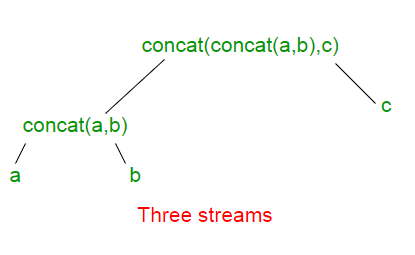GeeksforGeeks App
Open AppBrowser
Continue

# DoubleStream concat() in Java

DoubleStream concat() method creates a concatenated stream in which the elements are all the elements of the first stream followed by all the elements of the second stream. The resulting stream is ordered if both of the input streams are ordered, and parallel if either of the input streams is parallel.

Syntax :

```static DoubleStream concat(DoubleStream a,  DoubleStream b)
```

Parameters :

1. DoubleStream : A sequence of primitive double-valued elements.
2. a : Represents the first stream.
3. b : Represents the second stream.

Return Value : The function returns the concatenation of the two input DoubleStreams.

The calls to DoubleStream.concat(DoubleStream a, DoubleStream b) can be think of as forming a binary tree. The concatenation of all the input streams is at the root. The individual input streams are at the leaves. Below given is example for 3 DoubleStreams a, b and c.Each additional input stream adds one layer of depth to the tree and one layer of indirection to reach all the other streams.

Note : The elements returned by DoubleStream.concat() method is ordered. For example, the following two lines returns the same result:

```DoubleStream.concat(DoubleStream.concat(stream1, stream2), stream3);
DoubleStream.concat(stream1, DoubleStream.concat(stream2, stream3));
```

But the result for the following two are different.

```DoubleStream.concat(DoubleStream.concat(stream1, stream2), stream3);
DoubleStream.concat(DoubleStream.concat(stream2, stream1), stream3);
```

Example 1 :

 `// Implementation of DoubleStream.concat()``// method in Java 8 with 2 DoubleStreams``import` `java.util.*;``import` `java.util.stream.DoubleStream;``import` `java.util.stream.Stream;`` ` `class` `GFG {`` ` `    ``// Driver code``    ``public` `static` `void` `main(String[] args)``    ``{``        ``// Creating two DoubleStreams``        ``DoubleStream stream1 = DoubleStream.of(``2.2``, ``4.3``, ``6.4``);``        ``DoubleStream stream2 = DoubleStream.of(``1.5``, ``3.6``, ``5.7``);`` ` `        ``// concatenating both the Streams``        ``// with DoubleStream.concat() function``        ``// and displaying the result``        ``DoubleStream.concat(stream1, stream2)``            ``.forEach(element -> System.out.println(element));``    ``}``}`

Output:

```2.2
4.3
6.4
1.5
3.6
5.7
```

Example 2 :

 `// Implementation of DoubleStream.concat()``// method in Java 8 with 2 DoubleStreams``import` `java.util.*;``import` `java.util.stream.DoubleStream;``import` `java.util.stream.Stream;`` ` `class` `GFG {`` ` `    ``// Driver code``    ``public` `static` `void` `main(String[] args)``    ``{``        ``// Creating two DoubleStreams``        ``DoubleStream stream1 = DoubleStream.of(``2.2``, ``4.3``, ``6.4``);``        ``DoubleStream stream2 = DoubleStream.of(``1.5``, ``4.3``, ``2.2``);`` ` `        ``// concatenating both the Streams``        ``// with DoubleStream.concat() function``        ``// and displaying the result``        ``DoubleStream.concat(stream1, stream2).distinct().forEach(element -> System.out.println(element));``    ``}``}`

Output:

```2.2
4.3
6.4
1.5
```

My Personal Notes arrow_drop_up
Related Tutorials